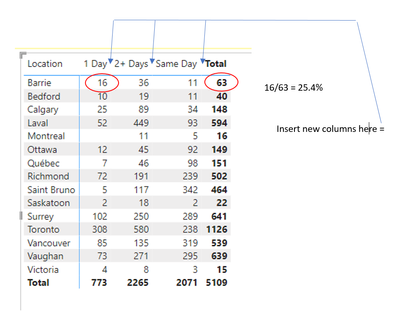cancel
Showing results for
Did you mean:Regular Visitor

## Divide values of one column by the total of a different column

I would like to add columns that take different subtotals for one location and divide by the total for that location in the format of percentage. See images for dataset and example2 REPLIES 2Resolver IV

Try creating a new measure as below, and adding as a second Values field in your matrix table:

``````Percentage =

DIVIDE(
CALCULATE(COUNT('BAML Checks'[Location]),
ALLEXCEPT('BAML Checks','BAML Checks'[Location],'BAML Checks'[Age Bucket]),
CALCULATE(COUNT('BAML Checks'[Location]),
ALLEXCEPT('BAML Checks','BAML Checks'[Location]),
0)``````

I don't think there is a way to hide the total % in a matrix table without hiding the count total, so you will end up with a % total of 100% for each row. If that's an issue you might need to build this using a standard Table visual with measures for each column.Super User

@simteron , for that you to have add an additional row to your dimension or use an extended dimension

Check how custom totals added here

Power BI How to get the P&L formatting right: https://youtu.be/C9K8uVfthUU

https://medium.com/microsoft-power-bi/power-bi-formatted-p-l-with-custom-sub-totals-and-blank-rows-e...